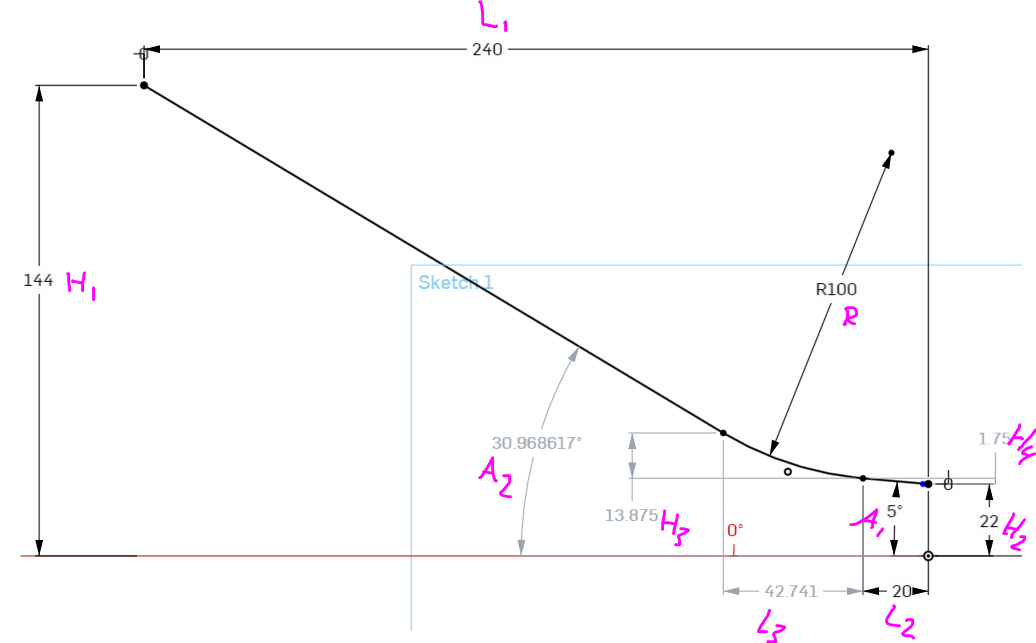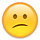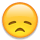Welcome to the Onshape forum! Ask questions and join in the discussions about everything Onshape.

First time visiting? Here are some places to start:

1. Looking for a certain topic? Check out the categories filter or use Search (upper right).
2. Need support? Ask a question to our Community Support category.
3. Please submit support tickets for bugs but you can request improvements in the Product Feedback category.
4. Be respectful, on topic and if you see a problem, Flag it.

If you would like to contact our Community Manager personally, feel free to send a private message or an email.

# Math Question (Finding an equation)

edited November 2019 in General
Non-Onshape question, but I figure there are enough smart people here, someone could help meI've always just had a CAD program available to me, I just let the software do the math. Silly me..

I have to make an excel sheet that can help quote some straight chutes. They can enter all the black numbers you see below in the form of variables, so I need the full equations to solve for the grey numbers.
The problem I have is I don't know how to solve for H₃ and L₃ which I need to ultimately find A₂

At the very least I need A₂ that is the number all of this mess is trying to solve for. It lets us know if packages will even slide down the chute, or if we need to add/remove friction surfaces.
H₃ and L₃ will help them visualize how large the swoop is without asking for a model.

Here is what I got so far:

H₃ = ? = ~13.875
H₄ = sin(A₁)L₂ = sin(5°)20 = 0.08716 * 20 = ~1.74311
L₃ = ? = ~42.741
A₂ = atan( (H₁-H₂-H₃-H₄) / (L₁-L₂-L₃) ) = atan( (144-22-13.875-1.74311) / (240-20-42.741) ) = ~30.97

Furthermore: the values of A₁ L₂ & H₄ can be equal to ZeroTagged:

• edited November 2019 Accepted Answer
Thank you @konstantin_shiriazdanov I'm sure that works, but I was having a hard time simplifying it.me no that smartMorgan shared a doc with me and we worked it out there. Here is the result he came up with which I transposed into VBA for excel.

EDIT: Fixed bug in code
```Public Function AngleOfMainChute(HorizontalLength As Double, ExitHorizontalLength As Double, EntryElevation As Double, ExitElevation As Double, ExitAngle As Double, SwoopRadius As Double)
toDeg = 180 / Application.WorksheetFunction.Pi()
l1 = HorizontalLength
l2 = ExitHorizontalLength
h1 = EntryElevation
h2 = ExitElevation

h4 = Tan(a1) * l2
arcEndX = l1 - l2
arcEndY = h1 - h2 - h4
arcCPointX = arcEndX + Sin(a1) * r
arcCPointY = arcEndY - Cos(a1) * r
triHypot = Math.Sqr(arcCPointX ^ 2 + arcCPointY ^ 2)

hypotToMainLineAngle = Application.WorksheetFunction.Asin(r / triHypot)
hypotAngle = Math.Atn(arcCPointY / arcCPointX)

result = hypotAngle + hypotToMainLineAngle
AngleOfMainChute = result * toDeg
End Function```
Thank you everyone• Thanks, Give me time to digest that• Member, Moderator, Onshape Employees, Developers Posts: 1,381Philip Thomas - Onshape
• Yea I know right? I only get part way thru it before I go crosseyed.Trying to take it out of featurescript notaion and get it back to simple math.  In the end I need to code it in visual basic in excel
• edited November 2019
you can set this repeatable piece from denominators to its own variable, for example:
``Sqrt(Power(L1 - L2 + R*Sin(A1),2) + Power(-H1 + H2 + R*Cos(A1) + L2*Tan(A1),2))`L0 = `
• edited November 2019 Accepted Answer
Thank you @konstantin_shiriazdanov I'm sure that works, but I was having a hard time simplifying it.me no that smartMorgan shared a doc with me and we worked it out there. Here is the result he came up with which I transposed into VBA for excel.

EDIT: Fixed bug in code
```Public Function AngleOfMainChute(HorizontalLength As Double, ExitHorizontalLength As Double, EntryElevation As Double, ExitElevation As Double, ExitAngle As Double, SwoopRadius As Double)
toDeg = 180 / Application.WorksheetFunction.Pi()
l1 = HorizontalLength
l2 = ExitHorizontalLength
h1 = EntryElevation
h2 = ExitElevation

h4 = Tan(a1) * l2
arcEndX = l1 - l2
arcEndY = h1 - h2 - h4
arcCPointX = arcEndX + Sin(a1) * r
arcCPointY = arcEndY - Cos(a1) * r
triHypot = Math.Sqr(arcCPointX ^ 2 + arcCPointY ^ 2)

hypotToMainLineAngle = Application.WorksheetFunction.Asin(r / triHypot)
hypotAngle = Math.Atn(arcCPointY / arcCPointX)

result = hypotAngle + hypotToMainLineAngle
AngleOfMainChute = result * toDeg
End Function```
Thank you everyone• edited November 2019
Forum bugged out, and made a this a separate post when I edited my comment to add a photo... Which I could delete a comment